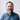# Binary to Decimal Conversion in Ruby

This week I wrote a small algorithm in Ruby to convert binary numbers into decimal numbers. Here’s the problem description, from Exercism.io:

Convert a binary number, represented as a string (e.g. ‘101010’), to its decimal equivalent using first principles. Implement binary to decimal conversion. Given a binary input string, your program should produce a decimal output. The program should handle invalid inputs.

And here is my code:

``````class Binary
def self.to_decimal(string)
raise ArgumentError if string.match?(/[^01]/)

string.reverse.chars.map.with_index do |digit, index|
digit.to_i * 2**index
end.sum
end
end``````

What’s going on here? Examining each line:

1. We make a class `Binary`, a container for our code.
2. We define a class method. Prefer functional when object instantiation isn’t beneficial.
3. Guard clause! Raise an `ArgumentError` if the string does not match the binary format we expect.
4. Space (let that code breathe).
5. Reverse the string and split into characters, then map over each with index included.
6. Take each digit (starting with the rightmost because we’ve reversed the string), convert it into a number, and multiply that number by two to the power of its enumerable index. In simpler terms, convert each digit to its binary value.
7. Sum the result; close out the function and the class.

### What’s Good

I like that my solution matches for `ArgumentError` positively, covering a range of bad inputs not specified by the test cases. I like that it reverses the string so that `index` can be used as the incrementing exponent. And, I like that it uses `.map` to return a result without an accumulator variable.

### What I’d Do Differently Next Time

I do not love that my solution takes an argument called `string`. Although that’s the type the method expects, it doesn’t tell the reader a lot about what this method does. If I could rewrite it, I’d name that variable something like `binary`.

My solution is also a bit clever; I may have golfed this down a little too much. In general, I think mathematical functions can get away with terser syntax than ordinary functions, because the mathematical principles are easy to learn about with a quick Google search.

### Conclusion

Thanks Exercism.io for this fun challenge, and thanks for reading! Here’s the permalink to my solution:

Binary to Decimal (Ruby)

Each week, I write an email about React. It's a collection of quotes, news, conference talks, and documentation curated be me to help you get up to speed on this exploding ecosystem. Join my subscribers today by subscribing to React Explained.